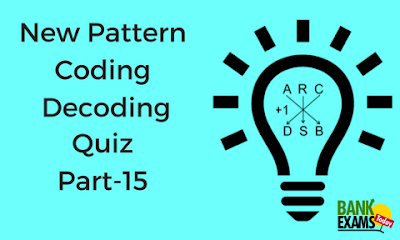New Students Offer - Use Code HELLO

# New Pattern Coding-Decoding Quiz Part-15Directions (Q.1-5): In a certain code language
"MIXED BUNCH SYSTEM GRAPES" is written as "@26B, @38I, #8R, #16F"
"HAND STOOL FINAL MEMBER" is written as "#24R, @8Z, #24G, @36V"
"SPEND FAST GOAL SUPER" is written as "#36F, @40Z, #8K, @24L"
"SERIAL RELAX FUNCTIONS EXAMS" is written as "@28F, #38C, @24V, #48V"

### Question .1

The code for the words ‘MOVIE TICKET’ is?
A. @20L, #24F
B. #10P, #38R
C. #10L, @40R
D. @24J, #12H
E. None of these
Ans. C.
Solution:
For Symbol:
If total numbers of word is even then we use @
If total numbers of word is odd then we use #
For Number = 2 × value of the last alphabet of the word
Letter = Opposite of the 2nd alphabet of the word
MOVIE TICKET = #10L, @40R

### Question .2

The code for the word ‘Happy’ is?
A. @40L
B. #50Z
C. @40Z
D. #50Y
E. None of these
Ans. B.
Solution:
For Symbol:
If total numbers of word is even then we use @
If total numbers of word is odd then we use #
For Number = 2 × value of the last alphabet of the word
Letter = Opposite of the 2nd alphabet of the word
Happy = #50Z

### Question .3

The code ‘@2N’ denotes which of the following word?
A. SIGNALS
B. LAPTOP
C. ZONE
D. UMBRELLA
E. EXAMS
Ans. D.
Solution:
For Symbol:
If total numbers of word is even then we use @
If total numbers of word is odd then we use #
For Number = 2 × value of the last alphabet of the word
Letter = Opposite of the 2nd alphabet of the word
UMBRELLA = @2N

### Question .4

What will be the code for the words 'SHE HAS MANY SWEETS'?
A. @10S, #40E, @26F, @38D
B. @24D, #38Z, @38R, #16G
C. #10S, #38Z, @50Z, @38D
D. #42G, @32T, @50Z, #38D
E. None of these
Ans. C.
Solution:
For Symbol:
If total numbers of word is even then we use @
If total numbers of word is odd then we use #
For Number = 2 × value of the last alphabet of the word
Letter = Opposite of the 2nd alphabet of the word
SHE HAS MANY SWEETS = #10S, #38Z, @50Z, @38D

### Question .5

What is the code for ‘FINAL’?
A. @40L
B. #24S
C. #18S
D. @26I
E. #24R
Ans. E.
Solution:
For Symbol:
If total numbers of word is even then we use @
If total numbers of word is odd then we use #
For Number = 2 × value of the last alphabet of the word
Letter = Opposite of the 2nd alphabet of the word
FINAL = #24R